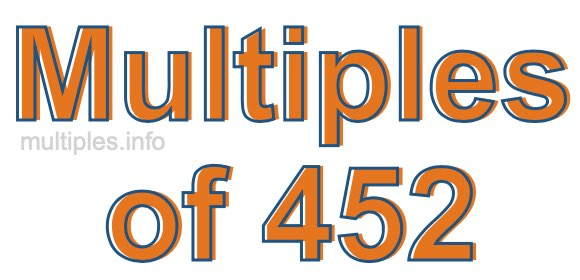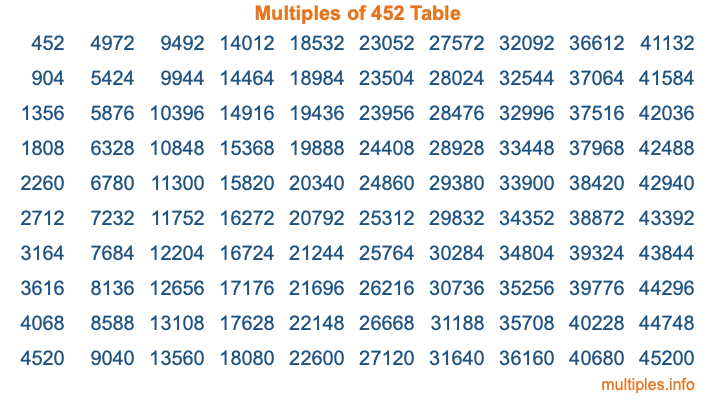Multiples of 452Welcome to the Multiples of 452 page. Here we will first teach you everything you will ever need to know about the multiples of 452, and then give you a study guide summary of everything we taught you to make sure you remember it all. Use this page to look up facts and learn information about the multiples of 452. This page will make you a multiples of four hundred fifty-two expert!

Definition of Multiples of 452
Multiples of 452 are all the numbers that when divided by 452 equal an integer. Each of the multiples of 452 are called a multiple. A multiple of 452 is created by multiplying 452 by an integer.

Therefore, to create a list of multiples of 452, you start with 1 multiplied by 452, then 2 multiplied by 452, then 3 multiplied by 452, and so on for as long as you want. Thus, the list of the first five multiples of 452 is 452, 904, 1356, 1808, and 2260. To see a larger list of multiples of 452, see the printable image of Multiples of 452 further down on this page. We also have a category where you can choose any nth multiple of 452.

Multiples of 452 Checker
The Multiples of 452 Checker below checks to see if any number of your choice is a multiple of 452. In other words, it checks to see if there is any number (integer) that when multiplied by 452 will equal your number. To do that, we divide your number by 452. If the the quotient is an integer, then your number is a multiple of 452.

Is  a multiple of 452?

Least Common Multiple of 452 and ...
A Least Common Multiple (LCM) is the lowest multiple that two or more numbers have in common. This is also called the smallest common multiple or lowest common multiple and is useful to know when you are adding our subtracting fractions. Enter one or more numbers below (452 is already entered) to find the LCM.

Check out our LCM Calculator if you need more details about the Least Common Multiple or if you need the LCM for different numbers for adding and subtraction fractions.

nth Multiple of 452
As we stated above, 452 is the first multiple of 452, 904 is the second multiple of 452, 1356 is the third multiple of 452, and so on. Enter a number below to find the nth multiple of 452.

th multiple of 452

Multiples of 452 vs Factors of 452
452 is a multiple of 452 and a factor of 452, but that is where the similarities end. All postive multiples of 452 are 452 or greater than 452. All positive factors of 452 are 452 or less than 452.

Below is the beginning list of multiples of 452 and the factors of 452 so you can compare:

Multiples of 452: 452, 904, 1356, 1808, 2260, etc.

Factors of 452: 1, 2, 4, 113, 226, 452

As you can see, the multiples of 452 are all the numbers that you can divide by 452 to get a whole number. The factors of 452, on the other hand, are all the whole numbers that you can multiply by another whole number to get 452.

It's also interesting to note that if a number (x) is a factor of 452, then 452 will also be a multiple of that number (x).

Multiples of 452 vs Divisors of 452
The divisors of 452 are all the integers that 452 can be divided by evenly. Below is a list of the divisors of 452.

Divisors of 452: 1, 2, 4, 113, 226, 452

The interesting thing to note here is that if you take any multiple of 452 and divide it by a divisor of 452, you will see that the quotient is an integer.

Multiples of 452 Table
Below is an image of the first 100 multiples of 452 in a table. The table is in chronological order, column by column. The first column has the first ten multiples of 452, the second column has the next ten multiples of 452, and so on.The Multiples of 452 Table is also referred to as the 452 Times Table or Times Table of 452. You are welcome to print out our table for your studies.

Negative Multiples of 452
Although not often discussed or needed in math, it is worth mentioning that you can make a list of negative multiples of 452 by multiplying 452 by -1, then by -2, then by -3, and so on, to get the following list of negative multiples of 452:

-452, -904, -1356, -1808, -2260, etc.

Multiples of 452 Summary
Below is a summary of important Multiples of 452 facts that we have discussed on this page. To retain the knowledge on this page, we recommend that you read through the summary and explain to yourself or a study partner why they hold true.

There are an infinite number of multiples of 452.

A multiple of 452 divided by 452 will equal a whole number.

452 divided by a factor of 452 equals a divisor of 452.

The nth multiple of 452 is n times 452.

The largest factor of 452 is equal to the first positive multiple of 452.

452 is a multiple of every factor of 452.

452 is a multiple of 452.

A multiple of 452 divided by a divisor of 452 equals an integer.

452 divided by a divisor of 452 equals a factor of 452.

Any integer times 452 will equal a multiple of 452.

Multiples of a Number
Here you can get the multiples of another number, all with the same attention to detail as we did for multiples of 452 on this page.

Multiples of
Multiples of 453
Did you find our page about multiples of four hundred fifty-two educational? Do you want more knowledge? Check out the multiples of the next number on our list!